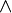# Logical conjunction facts for kids

Kids Encyclopedia Facts

Logical conjunction (very often called and) is a logic operation. It is represented by the symbol$\land$. Logical conjunction takes two truth values as inputs and returns an output. If both of the inputs are "true" at the same time, then logical conjunction outputs "true". If one of the inputs is false, or if both of the inputs are false, then logical conjunction outputs "false". Its logic gate equivalent is the AND gate.

## Related pagesLogical conjunction Facts for Kids. Kiddle Encyclopedia.